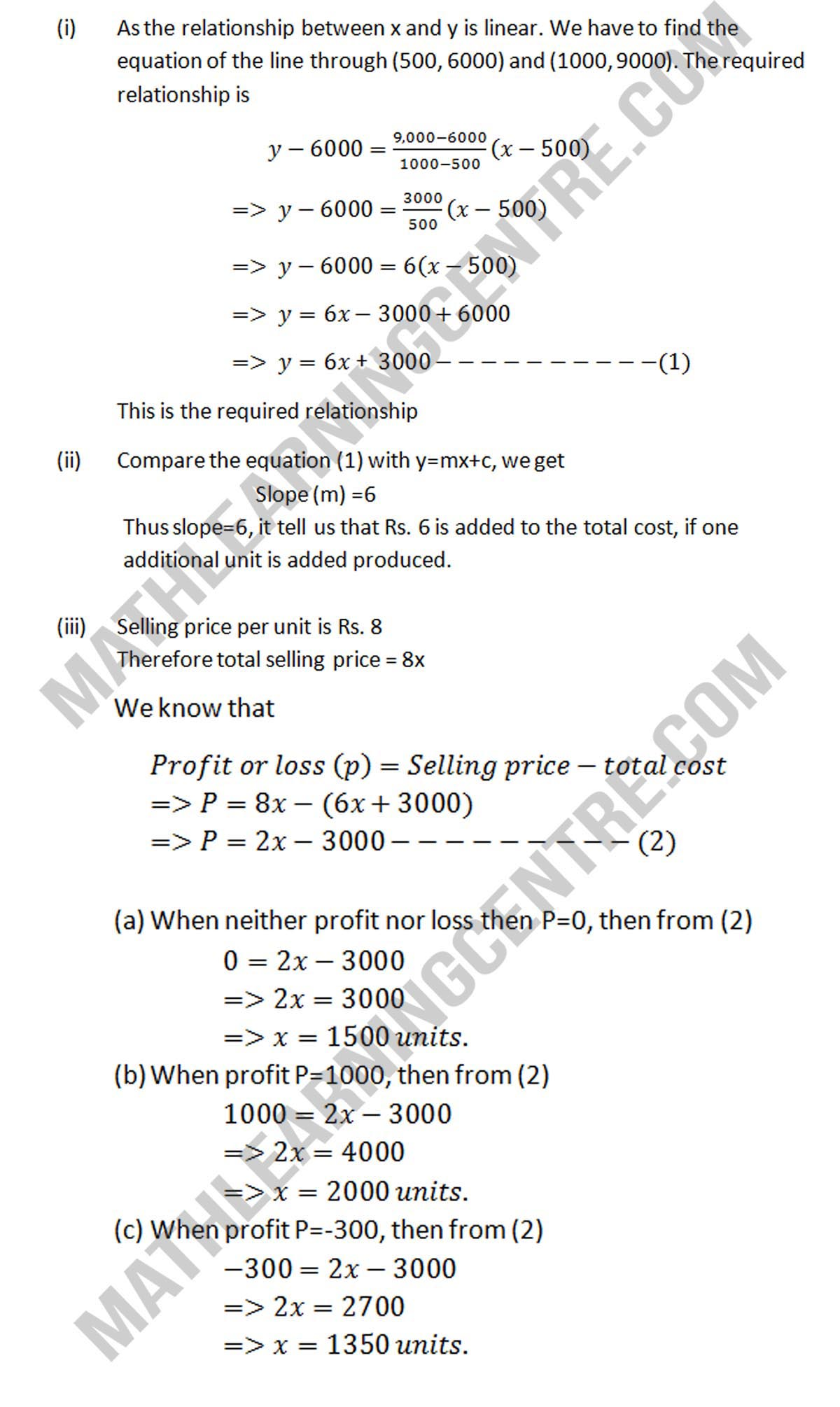# Coordinate Geometry

1. Find the linear relationship between x and y.
2. Find the slope of the line, what does it indicate
3. Find the number of units that must be produced so that
1. There is neither profit nor loss.
2. There is a profit of Rs. 1000
3. There is a loss of Rs. 300; it being given that the selling price is Rs. 8 per unit

## Solution